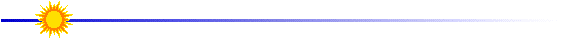# Tracking Satellites Using the Internet

Overview

Students studying physics use Internet resources to determine the orbital period of satellites.  Using Newton's Universal Law of Gravity, students then calculate satellite orbital velocities and altitudes. A printable version of this activity is found here.

1. Moving Stars?
2. Internet Tracking Maps
3. Part I - Determine the orbital period of Yohkoh
4. Part II - Determine the orbital velocity of Yohkoh
5. Part III - Determine the orbiting altitude of Yohkoh
6. Monitoring a Satellite Network

Learning Outcomes

By completing this activity, the learner will:

• view real-time maps showing satellite orbital paths on the Internet,
• estimate satellite orbital periods,
• calculate satellite orbital speeds, and
• determine satellite orbital alititudes.Lesson designed by the YPOP Team